# How to Apply the Same Filter to Multiple Sheets in Excel?

Filters can be applied to a single sheet in Excel, but applying the filter to multiple sheets at once is not possible by default. But we can make it happen with the help of the VBA application. Read this tutorial to learn how you can apply the same filter to multiple sheets in Excel. Applying a filter is simply gathering data based on a single entity.

## Applying the Same Filter to Multiple Sheets

In this case, we'll insert a VBA module and then run the code to apply filer. Let us see a simple process to understand how we can apply the same filter to multiple sheets in Excel using the VBA application.

Step 1

Let us consider a workbook that contains multiple worksheets; then, the data is similar to the data shown in the below image. We will be doing the process for two sheets, but we can apply the same process to any number of sheets in a single sheet.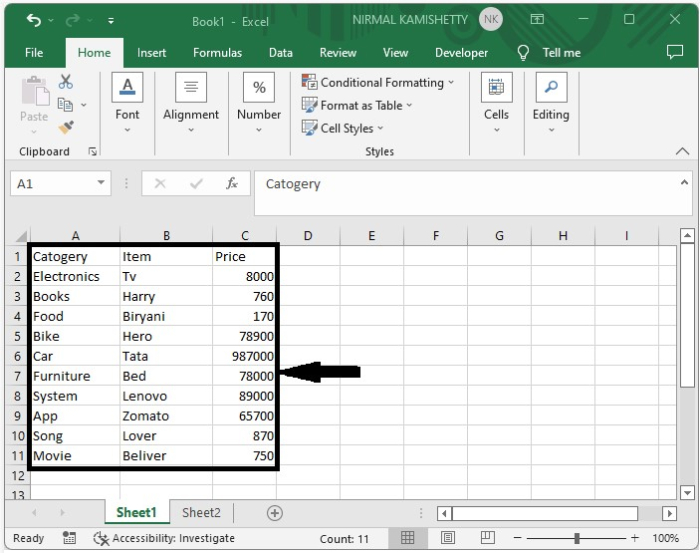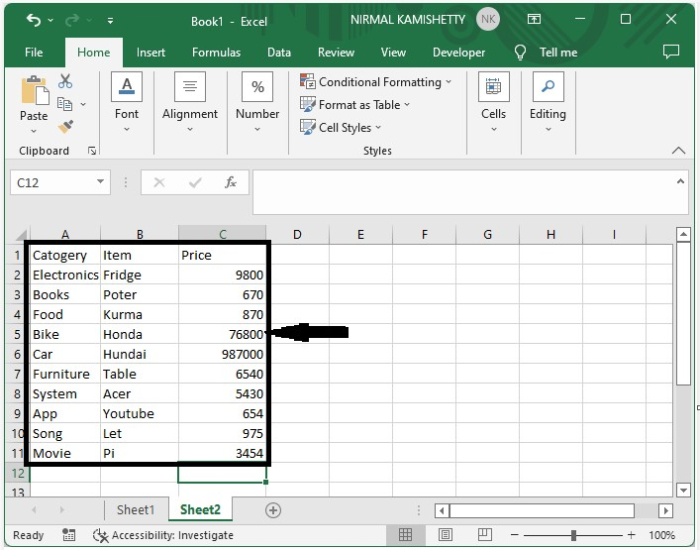Now, right-click on the sheet name and select view code to open the VBA application, then click on insert and select module, then type the programme into the textbox as shown in the below image.

### Program

Sub apply_autofilter_across_worksheets()
'Updateby Nirmal
Dim xWs As Worksheet
On Error Resume Next
For Each xWs In Worksheets
xWs.Range("A1").AutoFilter 1, "=Books"
Next
End Sub


In the above code, "A1" is the column, and "=Books" is the item you want to apply the filter based on.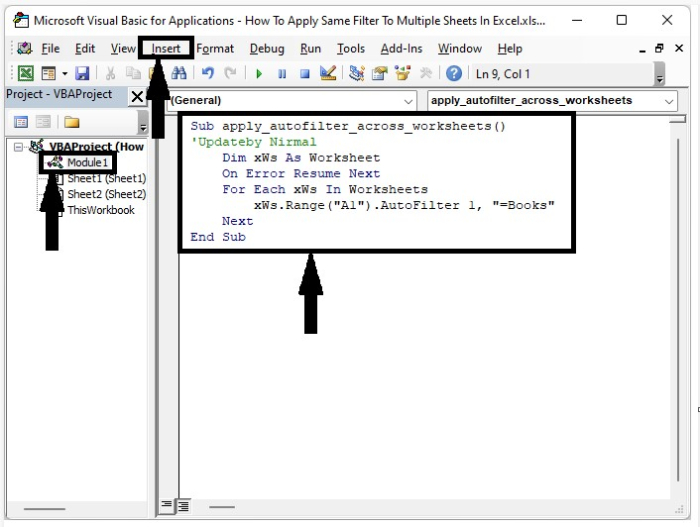Step 2

Now save the file as a macro-enabled template and click on F5 to run the code and successfully complete our process. Our final output will be similar to the data shown in the below image.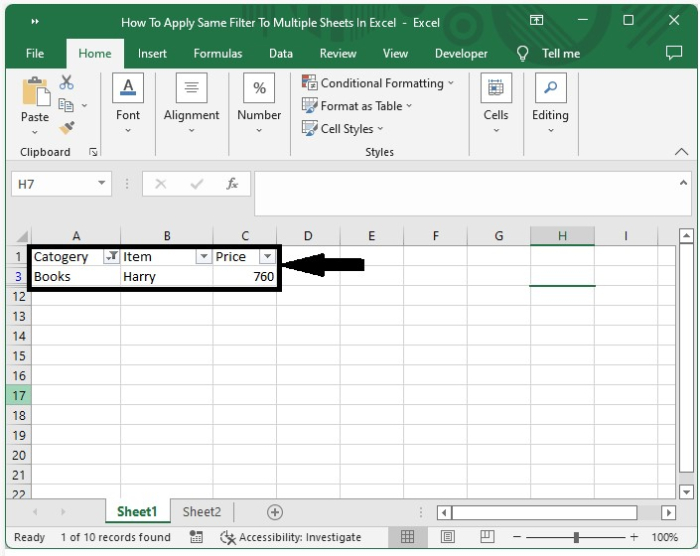Now, let's check the second sheet and see how it appears −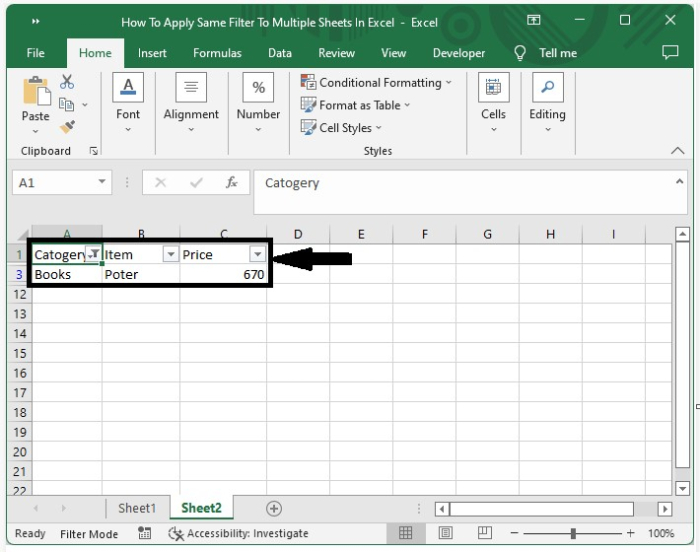## Conclusion

In this tutorial, we used a simple example to demonstrate how we can apply filters to multiple sheets in Excel to highlight a particular set of data.

Updated on: 03-Jan-2023

6K+ Views